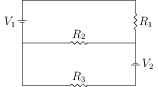# Kirchhoff's Laws and Simple Applications

## Problems from IIT JEE

Problem (IIT JEE 2014):Two ideal batteries of emf $V_1$ and $V_2$ and three resistances $R_1$, $R_2$ and $R_3$ are connected as shown in the figure. The current in resistance $R_2$ would be zero if,

1. $V_1=V_2$ and $R_1=R_2=R_3$
2. $V_1=V_2$ and $R_1=2R_2=R_3$
3. $V_1=2V_2$ and $2R_1=2R_2=R_3$
4. $2V_1=V_2$ and $2R_1=R_2=R_3$

Solution:Let $i_1$ and $i_2$ be the current as shown in figure. Apply Kirchhoff's law in the loop ABCDA and CEFDC to get, \begin{alignat}{2} \label{twb:eqn:1} & i_1R_1+(i_1-i_2)R_2=V_1,\\ \label{twb:eqn:2} & i_2R_3-(i_1-i_2)R_2=V_2. \end{alignat} Multiply first equation by $R_3$ and second equation by $R_1$ and then subtract to get current through $R_2$ as, \begin{alignat}{2} (i_1-i_2)=\frac{V_1 R_3-V_2 R_1}{R_1R_3+R_2R_3-R_1R_2}. \nonumber \end{alignat} The current through $R_2$ becomes zero when $V_1 R_3=V_2 R_1$. Thus, correct options are A, B, and D.